On The DO Loop blog, I write about a diverse set of topics, including statistical data analysis, machine learning, statistical programming, data visualization, simulation, numerical analysis, and matrix computations. In a previous article, I presented some of my most popular blog posts from 2020. The most popular articles often deal with elementary or familiar topics that are useful to almost every data analyst.

However, among last year's 100+ articles are many that discuss advanced topics. Did you make a New Year's resolution to learn something new this year? Here is your chance! The following articles were fun to write and deserve a second look.

### Machine learning concepts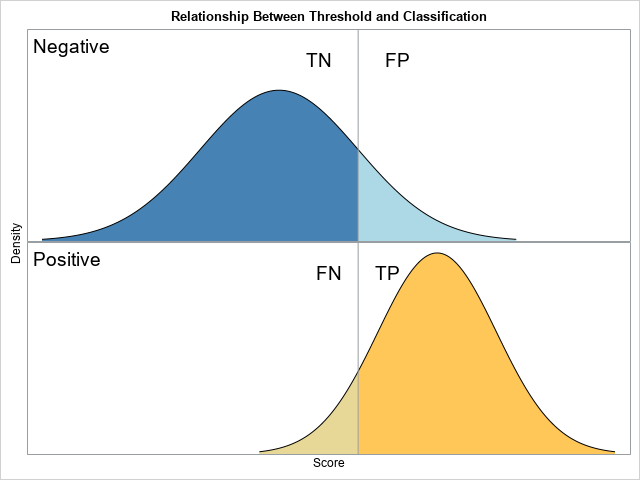Relationship between a threshold value and true/false negatives and positives

### Statistical smoothers

I write a lot about scatter plot smoothers, which are typically parametric or nonparametric regression models. But a SAS customer wanted to know how to get SAS to perform various classical interpolation schemes such as linear and cubic interpolations:

### SAS Viya and parallel computing

SAS is devoting tremendous resources to SAS Viya, which offers a modern analytic platform that runs in the cloud. One of the advantages of SAS Viya is the opportunity to take advantage of distributed computational resources. In 2020, I wrote a series of articles that demonstrate how to use the iml action in Viya 3.5 to implement custom parallel algorithms that use multiple nodes and threads on a cluster of machines. Whereas many actions in SAS Viya perform one and only one task, the iml action supports a general framework for custom, user-written, parallel computations:

• The map-reduce paradigm is a two-step process for distributing a computation. Every thread runs a function and produces a result for the data that it sees. The results are aggregated and returned. The iml action supports the MAPREDUCE function, which implements the map-reduce paradigm.
• The parallel-tasks paradigm is a way to run independent computations concurrently. The iml action supports the PARTASKS function, which implements the map-reduce paradigm.

### Simulation and visualization

Did I omit one of your favorite blog posts from The DO Loop in 2020? If so, leave a comment and tell me what topic you found interesting or useful. And if you missed some of these articles when they were first published, consider subscribing to The DO Loop in 2021.

The post Blog posts from 2020 that deserve a second look appeared first on The DO Loop.My 2020 SAS Global Forum paper was about how to write custom parallel programs by using the iml action in SAS Viya 3.5. My conference presentation was canceled because of the coronavirus pandemic, but I recently recorded a 15-minute video that summarizes the main ideas in the paper.

One of the reasons I enjoy attending conferences is to obtain a high-level "mental roadmap" of a topic that I can leverage if I decide to study the details. Hopefully, this video will provide you with a roadmap of the iml action and its capabilities.

If your browser does not support embedded video, you can go directly to the video on YouTube.

The video introduces a few topics that I have written about in more detail:

For more details you can read the paper: Wicklin and Banadaki, 2020, "Write Custom Parallel Programs by Using the iml Action."

The post Video: How to Write a Custom Parallel Program in SAS Viya appeared first on The DO Loop.I recently showed how to use simulation to estimate the power of a statistical hypothesis test. The example (a two-sample t test for the difference of means) is a simple SAS/IML module that is very fast. Fast is good because often you want to perform a sequence of simulations over a range of parameter values to construct a power curve. If you need to examine 100 sets of parameter values, you would expect the full computation to take about 100 times longer than one simulation.

But that calculation applies only when you run the simulations sequentially. If you run the simulations in parallel, you can complete the simulation study much faster. For example, if you have access to a machine or cluster of machines that can run 32 threads, then each thread needs to run only a few simulations. You can perform custom parallel computations by using the iml action in SAS Viya. The iml action is supported in SAS Viya 3.5.

This article shows how to distribute computations by using the PARTASKS function in the iml action. (PARTASKS stands for PARallel TASKS.) If you are not familiar with the iml action or with parallel processing, see my previous example that uses the PARTASKS function.

### Use simulation to estimate a power curve

I previously showed how to use simulation to estimate the power of a statistical test. In that article, I simulated data from N(15,5) and N(16,5) distributions. Because the t test is invariant under centering and scaling operations, this study is mathematically equivalent to simulating from the N(0,1) and N(0.2,1) distributions. (Subtract 15 and divide by 5.)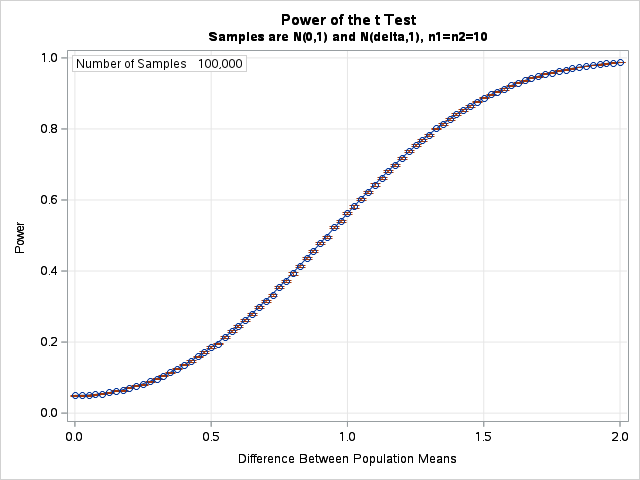Recall that the power of a statistical hypothesis test is the probability of making a Type 2 error. A Type 2 error is rejecting the null hypothesis when the alternative hypothesis is true. The power depends on the sample sizes and the magnitude of the effect you are trying to detect. In this case, the effect is the difference between two means. You can study how the power depends on the mean difference by estimating the power for a t test between data from an N(0,1) distribution and an N(δ, 1) distribution, where δ is a parameter in the interval [0, 2]. All hypothesis tests in this article use the α=0.05 significance level.

The power curve we want to estimate is shown to the right. The horizontal axis is the δ parameter, which represents the true difference between the population means. Each point on the curve is an estimate of the power of a two-sample t test for random samples of size n1 = n2 = 10. The curve indicates that when there is no difference between the means, you will conclude otherwise in 5% of random samples (because α=0.05). For larger differences, the probability of detecting the difference increases. If the difference is very large (more than 1.5 standard deviations apart), more than 90% of random samples will correctly reject the null hypothesis in the t test.

The curve is calculated at 81 values of δ, so this curve is the result of running 81 independent simulations. Each simulation uses 100,000 random samples and carries out 100,000 t tests. Although it is hard to see, the graph also shows 95% confidence intervals for power. The confidence intervals are very small because so many simulations are run.

So how long did it take to compute this power curve, which is the result of 8.1 million t tests? About 0.4 seconds by using parallel computations in the iml action.

Here's how you can write a program in the iml action to run 81 simulations across 32 threads. The following program uses four nodes, each with 8 threads, but you can achieve similar results by using other configurations. The main parts of the program are as follows:

• The program assumes that you have used the CAS statement in SAS to establish a CAS session that uses four worker nodes, each with at least eight CPUs. For example, the CAS statement might look something like this:
cas sess4 host='your_host_name' casuser='your_username' port=&MPPPort nworkers=4;
The IML program is contained between the SOURCE/ENDSOURCE statements in PROC CAS.
• The TTestH0 function implements the t test. It runs a t test on the columns of the input matrices and returns the proportion of samples that reject the null hypothesis. The TTestH0 function is explained in a previous article.
• The "task" we will distribute is the SimTTest function. (This encapsulates the "main program" in my previous article.) The SimTTest function simulates the samples, calls the TTestH0 function, and returns the number of t tests that reject the null hypothesis. The first sample is always from the N(0, 1) distribution and the second sample is from the N(&delta, 1) distribution. Each call creates B samples. The input to the SimTTest function is a list that contains all parameters: a random-number seed, the sample sizes (n1 and n2), the number of samples (B), and the value of the parameter (delta).
• The main program first sets up a list of arguments for each task. The i_th item in the list is the argument to the i_th task. For this computation, all arguments are the same (a list of parameters) except that the i_th argument includes the parameter value delta[i], where delta is a vector of parameter values in the interval [0, 2].
• The call to the PARTASKS function is
RL = ParTasks('SimTTest', ArgList, {2, 0});
where the first parameter is the task (or list of tasks), the second parameter is the list of arguments to the tasks, and the third parameter specifies how to distribute the computations. The documentation of the PARTASKS function provides the full details.
• After the PARTASKS function returns, the program processes the results. For each value of the parameter, δ, the main statistic is the proportion of t tests (p) that reject the null hypothesis. The program also computes a 95% (Wald) confidence interval for the binomial proportion. These statistics are written to a CAS table called 'PowerCurve' by using the MatrixWriteToCAS subroutine.
```/* Power curve computation: delta = 0 to 2 by 0.025 */ proc cas; session sess4; /* use session with four workers */ loadactionset 'iml'; /* load the iml action set */ source TTestPower;   /* Helper function: Compute t test for each column of X and Y. X is (n1 x m) matrix and Y is (n2 x m) matrix. Return the number of columns for which t test rejects H0 */ start TTestH0(x, y); n1 = nrow(X); n2 = nrow(Y); /* sample sizes */ meanX = mean(x); varX = var(x); /* mean & var of each sample */ meanY = mean(y); varY = var(y); poolStd = sqrt( ((n1-1)*varX + (n2-1)*varY) / (n1+n2-2) );   /* Compute t statistic and indicator var for tests that reject H0 */ t = (meanX - meanY) / (poolStd*sqrt(1/n1 + 1/n2)); t_crit = quantile('t', 1-0.05/2, n1+n2-2); /* alpha = 0.05 */ RejectH0 = (abs(t) > t_crit); /* 0 or 1 */ return RejectH0; /* binary vector */ finish;   /* Simulate two groups; Count how many reject H0: delta=0 */ start SimTTest(L); /* define the mapper */ call randseed(L\$'seed'); /* each thread uses different stream */ x = j(L\$'n1', L\$'B'); /* allocate space for Group 1 */ y = j(L\$'n2', L\$'B'); /* allocate space for Group 2 */ call randgen(x, 'Normal', 0, 1); /* X ~ N(0,1) */ call randgen(y, 'Normal', L\$'delta', 1); /* Y ~ N(delta,1) */ return sum(TTestH0(x, y)); /* count how many reject H0 */ finish;   /* ----- Main Program ----- */ numSamples = 1e5; L = [#'delta' = ., #'n1' = 10, #'n2' = 10, #'seed' = 321, #'B' = numSamples];   /* Create list of arguments. Each arg gets different value of delta */ delta = T( do(0, 2, 0.025) ); ArgList = ListCreate(nrow(delta)); do i = 1 to nrow(delta); L\$'delta' = delta[i]; call ListSetItem(ArgList, i, L); end;   RL = ParTasks('SimTTest', ArgList, {2, 0}); /* assign nodes before threads */   /* Summarize results and write to CAS table for graphing */ varNames = {'Delta' 'ProbEst' 'LCL' 'UCL'}; /* names of result vars */ Result = j(nrow(delta), 4, .); zCrit = quantile('Normal', 1-0.05/2); /* zCrit = 1.96 */ do i = 1 to nrow(delta); /* for each task */ p = RL\$i / numSamples; /* get proportion that reject H0 */ SE = sqrt(p*(1-p) / L\$'B'); /* std err for binomial proportion */ LCL = p - zCrit*SE; /* 95% CI */ UCL = p + zCrit*SE; Result[i,] = delta[i] || p || LCL || UCL; end;   call MatrixWriteToCas(Result, '', 'PowerCurve', varNames); endsource; iml / code=TTestPower nthreads=8; quit;```

You can pull the 81 statistics back to a SAS data set and use PROC SGPLOT to plot the results. You can download the complete program that generates the statistics and graphs the power curve.

### Summary

In summary, if you have a series of independent tasks in IML, you can use the PARTASKS function to distribute tasks to available threads. The speedup can be dramatic; it depends on the time required for the thread that performs the most work.

This article shows an example of using the PARTASKS function in the iml action, which is available in SAS Viya 3.5. The example shows how to distribute a sequence of computations among k threads that run concurrently and independently. In this example, k=32. Because the tasks are essentially identical, each thread computes 2/32 or 3/32 of the total work. The results from each task are returned in a list, where they can be further processed by the main program.

For more information and examples, see Wicklin and Banadaki (2020), "Write Custom Parallel Programs by Using the iml Action," which is the basis for these blog posts. Another source is the SAS IML Programming Guide, which includes documentation and examples for the iml action.

The post Estimate a power curve in parallel in SAS Viya appeared first on The DO Loop.The iml action was introduced in Viya 3.5. As shown in a previous article, the iml action supports ways to implement the map-reduce paradigm, which is a way to distribute a computation by using multiple threads. The map-reduce paradigm is ideal for “embarrassingly parallel” computations, which are composed of many independent and essentially identical computations. I wrote an example program that shows how to run a Monte Carlo simulation in parallel by using the iml action in SAS Viya.

Suppose you have three tasks. The first takes 2 seconds to run, the next takes 0.5 seconds, and the third takes 1 second. If you run a set of tasks sequentially, the total time is the sum of the times for the individual tasks: 2 + 0.5 + 1 = 3.5 seconds. In contrast, if you run the tasks in parallel, the total time is the maximum of the individual times: 2 seconds. Running the tasks in parallel saves time, and the speed-up depends on the ratio of the largest time to the total time, which is 2/3.5 in this example. Thus, the time for the parallel computation is 57% of the time for the sequential computation.

In general, the largest speedup occurs when the tasks each run in about the same time. In that situation (ignoring overhead costs), the time reduction can be as much 1/k when you run k tasks in k threads.

Let's run three tasks sequentially, then run the same tasks in parallel. Suppose you have a large symmetric N x N matrix and you need to compute three things: the determinant, the matrix inverse, and the eigenvalues. The following call to PROC IML runs the three tasks sequentially. The TOEPLITZ function is used to create a large symmetric matrix.

```proc iml; N = 2000; A = toeplitz( (N:1)/100 ); /* N x N symmetric matrix */   det = det(A); /* get det(A) */ inv = inv(A); /* get inv(A) */ eigval = eigval(A); /* get eigval(A) */```

The previous computations are performed by directly calling three built-in SAS/IML functions. Of course, in practice, the tasks will be more complex than these. Almost surely, each task will be encapsulated into a SAS/IML module. To emulate that process, I am going to define three trivial "task modules." The following statements perform The same computations, but now each "task" is performed by calling a user-written module:

```start detTask(A); return det(A); finish; start invTask(A); return inv(A); finish; start eigvalTask(A); return eigval(A); finish;   det = detTask(A); /* get det(A) */ inv = invTask(A); /* get inv(A) */ eigval = eigvalTask(A); /* get eigval(A) */```

### The syntax of the PARTASKS function

You can use the PARTASKS function to run the three tasks concurrently. To use the PARTASKS function, each task must be a user-defined SAS/IML function that has exactly one input argument and returns one output argument. For our example, the detTask, invTask, and eigvalTask modules satisfy these requirements. (If a task requires more than one input argument, you can pack the arguments into a list and pass the list as a single argument.)

The syntax of the PARTASKS function is
where

• TaskArgs is a list of arguments. The i_th argument will be sent to the i_th module. In this example, each module takes the same matrix, so you can create a list that has three items, each being the same matrix: TaskArgs = [A, A, A];
• Options is an (optional) vector that specifies how the tasks are distributed. The documentation of the PARTASKS function gives the full details, but the first element of the vector specifies how to distribute the computation to nodes and threads, and the second element specifies whether to print information about the performance of the tasks. For this example, you can choose options = {1, 1};, which will distribute tasks to threads on the controller node and print information about the tasks.

Suppose you are running SAS Viya and you use the have access to a machine that has at least four threads. You can use the CAS statement to define a session that will use only one machine (no worker nodes). The CAS statement might look something like this:
cas sess0 host='your_host_name' casuser='your_username' port=&YourPort; /* SMP, 0 workers */
Then you can run the PARTASKS function by making the following call to the iml action. If you are not familiar with the iml action, see the getting started example for the iml action.

```proc cas; session sess0; /* SMP session: controller node only */ loadactionset 'iml'; /* load the action set */ source partasks; /* put program in SOURCE/ENDSOURCE block */ start detTask(A); /* 1st task */ return det(A); finish; start invTask(A); /* 2nd task */ return inv(A); finish; start eigvalTask(A); /* 3rd task */ return eigval(A); finish;   /* ----- Main Program ----- */ N = 2000; A = toeplitz( (N:1)/100 ); /* N x N symmetric matrix */ Tasks = {'detTask' 'invTask' 'eigvalTask'}; Args = [A, A, A]; /* each task gets same arg in this case */ opt = {1, /* distribute to threads on controller */ 1}; /* display information about the tasks */ Results = ParTasks(Tasks, Args, opt); /* results are returned in a list */   /* the i_th list item is the result of the i_th task */ det = Results\$1; /* get det(A) */ inv = Results\$2; /* get inv(A) */ eigval = Results\$3; /* get eigval(A) */ endsource; iml / code=partasks nthreads=4; run;```

The results are identical to the sequential computations in PROC IML. However, this program executes the three tasks in parallel, so the total time for the computations is the time required by the longest task.

### Visualize the parallel tasks computations

You can use the following diagram to help visualize the program. (Click to enlarge.)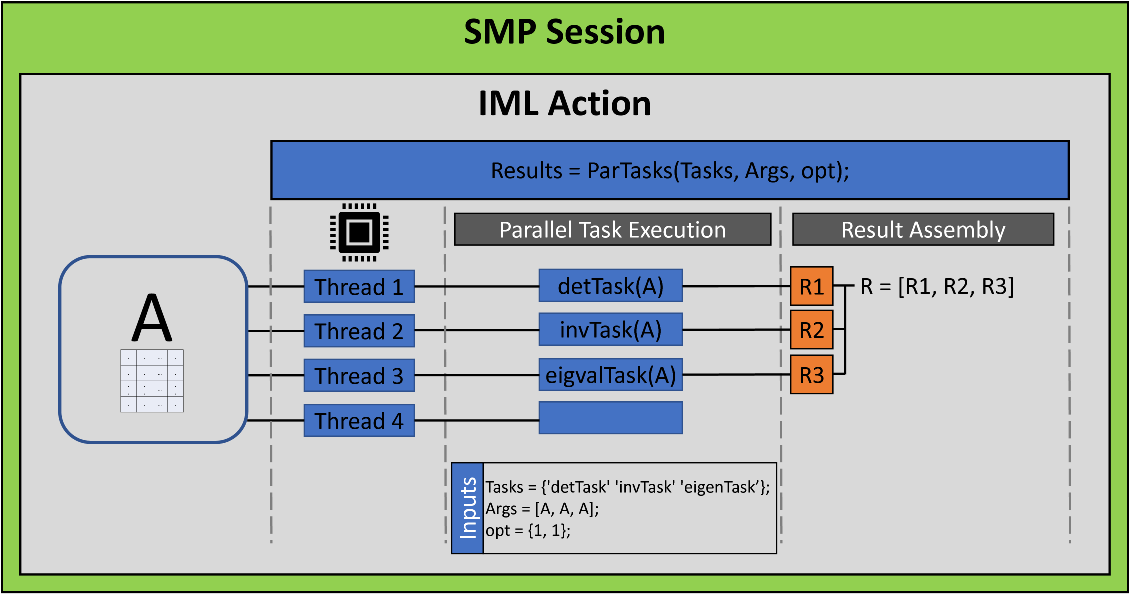The diagram shows the following:

• The CAS session does not include any worker nodes. Therefore, the iml action runs entirely on the controller, although it can still use multiple threads. This mode is known as symmetric multiprocessing (SMP) or single-machine mode. Notice that the call to the iml action (the statement just before the RUN statement) specifies the NTHREADS=4 parameter, which causes the action to use four threads. Because there are only three tasks, one thread remains idle.
• The program defines the matrix, A, the vector of module names, and the list of arguments. When you call the ParTasks function, the i_th argument is sent to the i_th module on the i_th thread.
• Each thread runs a different function module. Each function returns its result. The results are returned in a list. The i_th item in the list is the result of the call to the i_th function module.

### Summary

This article demonstrates a simple call to the PARTASKS function in the iml action, which is available in SAS Viya 3.5. The example shows how to distribute tasks among k threads. Each thread runs concurrently and independently. The total time for the computation depends on the longest time that any one thread spends on its computation.

This example is simple but demonstrates the main ideas of distributing tasks in parallel. In a future article, I will present a more compelling example.

For more information and examples, see Wicklin and Banadaki (2020), "Write Custom Parallel Programs by Using the iml Action," which is the basis for these blog posts. Another source is the SAS IML Programming Guide, which includes documentation and examples for the iml action.

The post Run tasks in parallel in SAS Viya appeared first on The DO Loop.A previous article introduces the MAPREDUCE function in the iml action. (The iml action was introduced in Viya 3.5.) The MAPREDUCE function implements the map-reduce paradigm, which is a two-step process for distributing a computation to multiple threads. The example in the previous article adds a set of numbers by distributing the computation among four threads. During the "map" step, each thread computes the partial sum of one-fourth of the numbers. During the "reduce" step, the four partial sums are added to obtain the total sum. The same map-reduce framework can be used to implement a wide range of parallel computations. This article uses the MAPREDUCE function in the iml action to implement a parallel version of a Monte Carlo simulation.

### Serial Monte Carlo simulation

Before running a simulation study in parallel, let's present a serial implementation in the SAS/IML language. In a Monte Carlo simulation, you simulate B random samples of size N from a probability distribution. For each sample, you compute a statistic. When B is large, the distribution of the statistics is a good approximation to the true sampling distribution for the statistic.

The example in this article uses simulation to approximate the sampling distribution of the sample mean. You can use the sampling distribution to estimate the standard error and to estimate a confidence interval for the mean. This example appears in the book Simulating Data with SAS(Wicklin 2013, p. 55–57) and in the paper "Ten Tips for Simulating Data with SAS" (Wicklin 2015, p. 6-9). The SAS/IML program runs a simulation to approximate the sampling distribution of the sample mean for a random sample of size N=36 that is drawn from the uniform U(0, 1) distribution. It simulates B=1E6 (one million) samples. The main function is the SimMeanUniform function, which does the following:

1. Uses the RANDSEED subroutine to set a random number seed.
2. Uses the RANDGEN subroutine to generate B samples of size N from the U(0, 1) distribution. In the N x B matrix, X, each column is a sample and there are B samples.
3. Uses the MEAN function to compute the mean of each sample (column) and returns the row vector of the sample means.

The remainder of the program estimates the population mean, the standard error of the sample mean, and a 95% confidence interval for the population mean.

```proc iml; start SimMeanUniform(N, seed, B); call randseed(seed); /* set random number stream */ x = j(N, B); /* allocate NxB matrix for samples */ call randgen(x, 'Uniform'); /* simulate from U(0,1) */ return mean(x); /* return row vector = mean of each column */ finish;   /* Simulate and return Monte Carlo distribution of mean */ stat = SimMeanUniform(36, /* N = sample size */ 123, /* seed = random number seed */ 1E6); /* B = total number of samples */   /* Monte Carlo estimates: mean, std err, and 95% CI of mean */ alpha = 0.05; stat = colvec(stat); numSamples = nrow(stat); MCEst = mean(stat); /* estimate of mean, */ SE = std(stat); /* standard deviation, */ call qntl(CI, stat, alpha/2 || 1-alpha/2); /* and 95% CI */ R = numSamples || MCEst || SE || CI`; /* combine for printing */ print R[format=8.4 L='95% Monte Carlo CI (Serial)' c={'NumSamples' 'MCEst' 'StdErr' 'LowerCL' 'UpperCL'}];```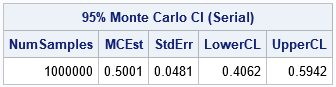This program takes less than 0.5 seconds to run. It simulates one million samples and computes one million statistics (sample means). More complex simulations take longer and can benefit by distributing the computation to many threads, as shown in the next section.

### A distributed Monte Carlo simulation

Suppose that you have access to a cluster of four worker nodes, each of which runs eight threads. You can distribute the simulation across the 32 threads and ask each thread to perform 1/32 of the simulation. Specifically, each thread can simulate 31,250 random samples from U(0,1) and return the sample means. The sample means can then be concatenated into a long vector and returned. The rest of the program (the Monte Carlo estimates) does not need to change.

The goal is to get the SimMeanUniform function to run on all 32 available threads. One way to do that is to use the SimMeanUniform function as the mapping function that is passed to the MAPREDUCE function. To use SimMeanUniform as a mapping function, you need to make two small modifications:

• The function currently takes three arguments: N, seed, and B. But a mapping function that is called by the MAPREDUCE function is limited to a single argument. The solution is to pack the arguments into a list. For example, in the main program define L = [#'N'=36, #'seed' = 123, #'B' = 1E6] and pass that list to the MAPREDUCE function. In the definition of the SimMeanUniform module, define the signature as SimMeanUniform(L) and access the parameters as L\$'N', L\$'seed', and L\$'B'.
• The SimMeanUniform function currently simulates B random samples. But if this function runs on 32 threads, we want each thread to generate B/32 random samples. One solution would be to explicitly pass in B=1E6/32, but a better solution is to use the NPERTHREAD function in the iml action. The NPERTHREAD function uses the FLOOR-MOD trick to determine how many samples should be simulated by each thread. You specify the total number of simulations, and the NPERTHREAD function uses the thread ID to determine the number of simulations for each thread.

With these two modifications, you can implement the Monte Carlo simulation in parallel in the iml action. Because the mapping function returns a row vector, the reducer will be horizontal concatenation, which is available as the built-in "_HCONCAT" reducer. Thus, although each thread returns a row vector that has 31,250 elements, the MAPREDUCE function will return a row vector that has one million elements. The following program implements the parallel simulation in the iml action. If you are not familiar with using PROC CAS to call the iml action, see the getting started example for the iml action.

```/* Simulate B independent samples from a uniform(0,1) distribution. Mapper: Generate M samples, where M ~ B/numThreads. Return M statistics. Reducer: Concatenate the statistics. Main Program: Estimate standard error and CI for mean. */ proc cas; session sess4; /* use session with four workers */ loadactionset 'iml'; /* load the action set */ source SimMean; /* put program in SOURCE/ENDSOURCE block */   start SimMeanUniform(L); /* define the mapper */ call randseed(L\$'seed'); /* each thread uses different stream */ M = nPerThread(L\$'B'); /* number of samples for thread */ x = j(L\$'N', M); /* allocate NxM matrix for samples */ call randgen(x, 'Uniform'); /* simulate from U(0,1) */ return mean(x); /* row vector = mean of each column */ finish;   /* ----- Main Program ----- */ /* Put the arguments for the mapper into a list */ L = [#'N' = 36, /* sample size */ #'seed' = 123, /* random number seed */ #'B' = 1E6 ]; /* total number of samples */ /* Simulate on all threads; return Monte Carlo distribution */ stat = MapReduce(L, 'SimMeanUniform', '_HCONCAT'); /* reducer is "horiz concatenate" */   /* Monte Carlo estimates: mean, std err, and 95% CI of mean */ alpha = 0.05; stat = colvec(stat); numSamples = nrow(stat); MCEst = mean(stat); /* estimate of mean, */ SE = std(stat); /* standard deviation, */ call qntl(CI, stat, alpha/2 || 1-alpha/2); /* and 95% CI */ R = numSamples || MCEst || SE || CI`; /* combine for printing */ print R[format=8.4 L='95% Monte Carlo CI (Parallel)' c={'NumSamples' 'MCEst' 'StdErr' 'LowerCL' 'UpperCL'}];   endsource; /* END of the SOURCE block */ iml / code=SimMean nthreads=8; /* run the iml action in 8 threads per node */ run;```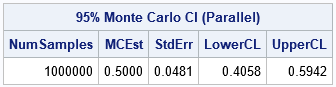The output is very similar to the output from PROC IML. There are small differences because this program involves random numbers. The random numbers used by the serial program are different from the numbers used by the parallel program. By the way, each thread in the parallel program uses an independent set of random numbers. In a future article. I will discuss generating random numbers in parallel computations.

Notice that the structure of the parallel program is very similar to the serial program. The changes needed to "parallelize" the serial program are minor for this example. The benefit, however, is huge: The parallel program runs about 30 times faster than the serial program.

### Summary

This article shows an example of using the MAPREDUCE function in the iml action, which is available in SAS Viya 3.5. The example shows how to divide a simulation study among k threads that run concurrently and independently. Each thread runs a mapping function, which simulates and analyzes one-kth of the total work. (In this article, k=32.) The results are then concatenated to form the final answer, which is a sampling distribution.

For comparison, the Monte Carlo simulation is run twice: first as a serial program in PROC IML and again as a parallel program in the iml action. Converting the program to run in parallel requires some minor modifications but results in a major improvement in overall run time.

For more information and examples, see Wicklin and Banadaki (2020), "Write Custom Parallel Programs by Using the iml Action," which is the basis for these blog posts. Another source is the SAS IML Programming Guide, which includes documentation and examples for the iml action.

The post A parallel implementation of Monte Carlo simulation in SAS Viya appeared first on The DO Loop.The iml action in SAS Viya (introduced in Viya 3.5) provides a set of general programming tools that you can use to implement a custom parallel algorithm. This makes the iml action different than other Viya actions, which use distributed computations to solve specific problems in statistics, machine learning, and artificial intelligence. By using the iml action, you can use programming statements to define the problem you want to solve. One of the simplest ways to run a parallel program is to use the MAPREDUCE function in the iml action, which enables you to distribute a computation across threads and nodes. This article describes the MAPREDUCE function and gives an example.

### What is the map-reduce paradigm?

The MAPREDUCE function implements the map-reduce paradigm, which is a two-step process for distributing a computation. The MAPREDUCE function runs a SAS/IML module (called the mapping function, or the mapper) on every available node and thread in your CAS session. Each mapping function returns a result. The results are aggregated by using a reducing function (the reducer). The final aggregated result is returned by the MAPREDUCE function. The MAPREDUCE function is ideal for “embarrassingly parallel” computations, which are composed of many independent and essentially identical computations. Examples in statistics include Monte Carlo simulation and resampling methods such as the bootstrap. A Wikipedia article about the map-reduce framework includes other examples and more details.

### A simple map-reduce example: Adding numbers

You can use the MAPREDUCE function in the iml action to implement the map-reduce paradigm. The syntax of the MAPREDUCE function is
result = MAPREDUCE( mapArg, 'MapFunc', 'RedFunc' );
In this syntax, 'MapFunc' is the name of the mapping function and mapArg is a parameter that is passed to the mapper function in every thread. The 'RedFunc' argument is the name of the reducing function. You can use a predefined (built-in) reducers, or you can define your own reducer. This article uses only predefined reducers.

Let's implement the sum-of-N-numbers algorithm in the iml action by using four threads to sum the numbers 1, 2, ..., 1000. (For simplicity, I chose N divisible by 4, so each thread sums N/4 = 250 numbers.) There are many ways to send data to the mapper. This program packs the data into a matrix that has four rows and tells each thread to analyze one row. The program defines a helper function (getThreadID) and a mapper function (AddRow), which will run on each thread. The AddRow function does the following:

1. Call the getThreadID function to find the thread in which the AddRow function is running. The getThreadID function is a thin wrapper around the NODEINFO function, which is a built-in function in the iml action. The thread ID is stored in the variable j.
2. Extract the j th row of numbers. These are the numbers that the thread will sum.
3. Call the SUM function to compute the partial sum. Return that value.

In the following program, the built-in '_SUM' reducer adds the partial sums and returns the total sum. The program prints the result from each thread. Because the computation is performed in parallel, the order of the output is arbitrary and can vary from run to run. If you are not familiar with using PROC CAS to call the iml action, see the getting started example for the iml action.

```/* assume SESS0 is a CAS session that has 0 workers (controller only) and at least 4 threads */ proc cas; session sess0; /* SMP session: controller node only */ loadactionset 'iml'; /* load the action set */ source MapReduceAdd; start getThreadID(j); /* this function runs on the j_th thread */ j = nodeInfo()\$'threadId'; /* put thread ID into the variable j */ finish; start AddRow(X); call getThreadId(j); /* get thread ID */ sum = sum(X[j, ]); /* compute the partial sum for the j_th row */ print sum; /* print partial sum for this thread */ return sum; /* return the partial sum */ finish;   /* ----- Main Program ----- */ x = shape(1:1000, 4); /* create a 4 x 250 matrix */ Total = MapReduce(x, 'AddRow', '_SUM'); /* use built-in _SUM reducer */ print Total; endsource; iml / code=MapReduceAdd nthreads=4; run;```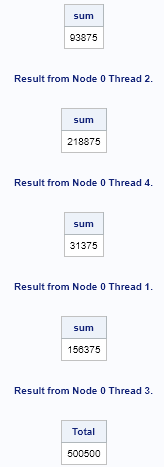The output shows the results of the PRINT statement in each thread. The output can appear in any order. For this run, the first output is from the second thread, which computes the sum of the numbers 251, 252, . . . , 500 and returns the value 93,875. The next output is from the fourth thread, which computes the sum of the numbers 751, 752, . . . , 1000. The other threads perform similar computations. The partial sums are sent to the built-in '_SUM' reducer, which adds them together and returns the total sum to the main program. The total sum is 500,500 and appears at the end of the output.

### Visualize the map-reduce computations

You can use the following diagram to help visualize the program. (Click to enlarge.)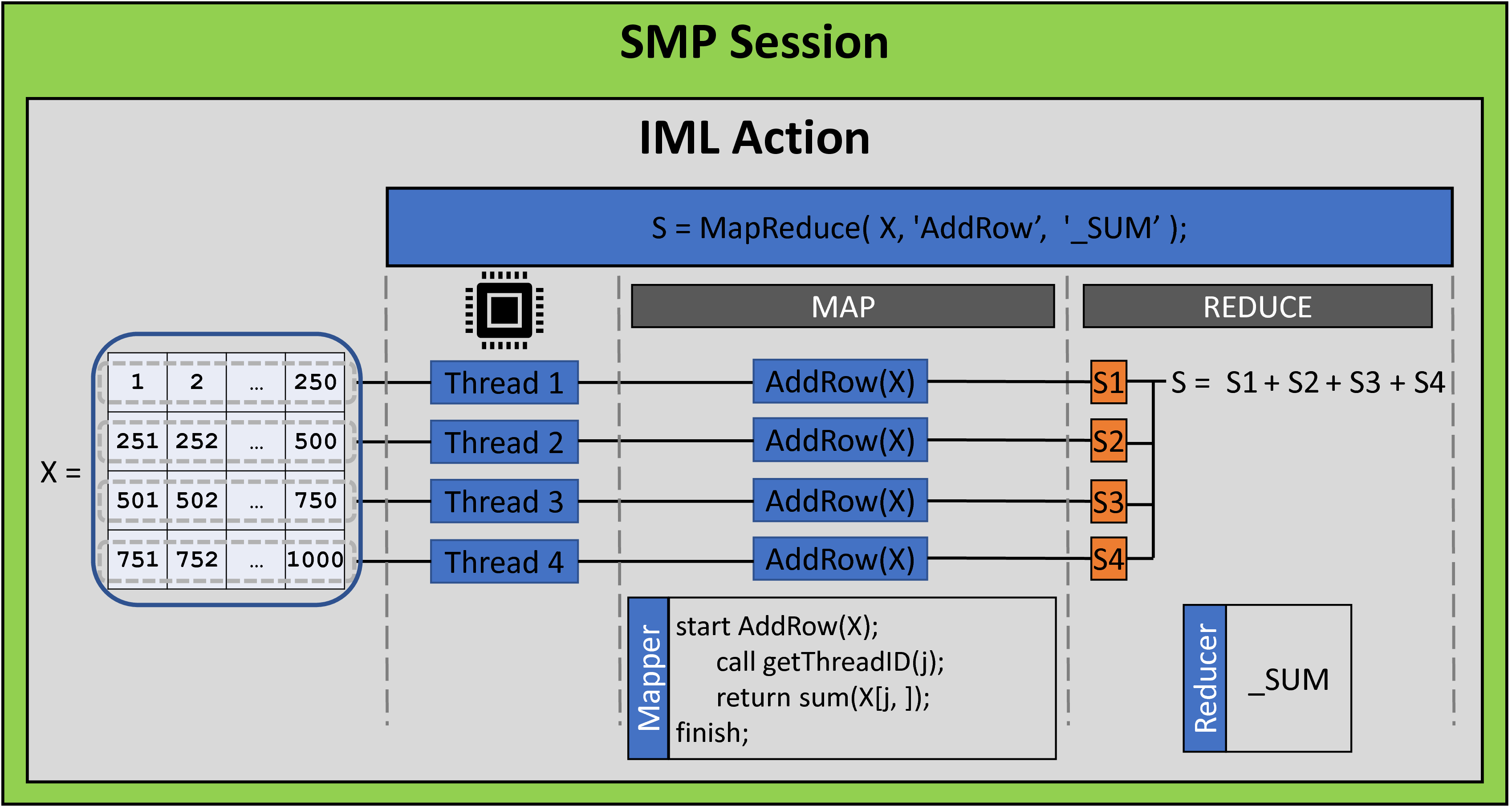The following list explains parts of the diagram:

• My CAS session did not include any worker nodes. Therefore, the iml action runs entirely on the controller, although it can still use multiple threads. This mode is known as symmetric multiprocessing (SMP) or single-machine mode. Notice that the call to the iml action (the statement just before the RUN statement) specifies the NTHREADS=4 parameter, which causes the action to use four threads.
• The program defines the matrix X, which has four rows. This matrix is sent to each thread.
• Each thread runs the AddRow function (the mapper). The function uses the NODEINFO function to determine the thread it is running in. It then sums the corresponding row of the X matrix and returns that partial sum.
• The reducer combines the results of the four mappers. In this case, the reducer is '_SUM', so the reducer adds the four partial sums. This sum is the value that is returned by the MAPREDUCE function.

### Summary

This example is one of the simplest map-reduce examples. Obviously, you do not need parallel computations to add a set of numbers, but I hope the simple example enables you to focus on map-reduce architecture. In a future article, I will present a more compelling example.

For more information and examples, see Wicklin and Banadaki (2020), "Write Custom Parallel Programs by Using the iml Action," which is the basis for these blog posts. Another source is the SAS IML Programming Guide, which includes documentation and examples for the iml action.

The post A general method for parallel computation in SAS Viya appeared first on The DO Loop.A previous article shows how to use the iml action to read a CAS data table into an IML matrix. This article shows how to write a CAS table from data in an IML matrix. You can read an overview of the iml action, which was introduced in SAS Viya 3.5.

### Write a CAS data table from the iml action

Suppose you are running a program in the iml action and you want to write the result of a computation to a CAS data table. The simplest way is to use the the MatrixWriteToCAS subroutine. The syntax of the function is
call MatrixWriteToCAS(matrix, caslib, TableName, colnames);
where

• matrix is the matrix that you want to save.
• caslib is a caslib that specifies the location of the table. A blank string means "use the default caslib." Otherwise, specify the name of a caslib. For example, if the CAS table is in your personal caslib, specify 'CASUSER(userName)', where userName is your login name. For more information, see the CAS documentation about caslibs.
• TableName is a string that specifies the name of the CAS table.
• colnames is a character vector, whose elements specify the columns in the CAS table.

For simplicity, the following call to the iml action defines a matrix and then writes it to a table. In practice, the matrix would be the result of some computation:

```proc cas; loadactionset 'iml'; /* load action set (once per session) */ source WriteMat; corr = {1.0000 0.7874 -0.7095 -0.7173 0.8079, 0.7874 1.0000 -0.6767 -0.6472 0.6308, -0.7095 -0.6767 1.0000 0.9410 -0.7380, -0.7173 -0.6472 0.9410 1.0000 -0.7910, 0.8079 0.6308 -0.7380 -0.7910 1.0000 }; varNames = {'EngineSize' 'Horsepower' 'MPG_City' 'MPG_Highway' 'Weight'}; call MatrixWriteToCas(corr, ' ', 'MyCorr', varNames); /* Write to CAS data table in default caslib */ endsource; iml / code=WriteMat; /* call the iml action to run the program */ run;```

The program writes the matrix to a CAS table named MYCORR. You can call the columnInfo action (which is similar to PROC CONTENTS) to verify that the CAS table exists:

```proc cas; columnInfo / table='MyCorr'; run;```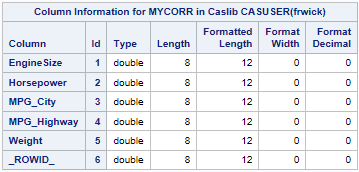The output of the columnInfo action shows that the MYCORR data table was created. Notice that in addition to the five specified variables, the CAS table contains a sixth variable, called _ROWID_. This variable is automatically added by the MatrixWriteToCAS subroutine.

How did that variable get there? As explained in the previous article, CAS data tables do not have an inherent row order, but matrices do. The iml action includes the _ROWID_ variable in the output data table in case you need to read the data back into an IML matrix at a later time. If you do, the rows of the new matrix will be in the same order as the rows of the CORR matrix that created the data table. For a correlation matrix, this is important because (by convention) the order of the rows corresponds to the order of the columns. Preserving the row order ensures that the matrix has 1s along the main diagonal.

In summary, you can use the MatrixWriteToCAS subroutine to write a matrix to a CAS table. Not only does the call create a CAS table, but it augments the table with information that can recreate the matrix in the same row order. By the way, if you want to save mixed-type data, you can use the TableWriteToCAS subroutine to write a SAS/IML table to a CAS table.

The post Write a CAS data table by using the iml action appeared first on The DO Loop.A previous article compares a SAS/IML program that runs in PROC IML to the same program that runs in the iml action. (You can read an overview of the iml action.) The example in the previous article was very simple and did not read or write data. This article compares a PROC IML program that reads a SAS data set to a similar program that runs in the iml action and reads CAS tables. The iml action was introduced in SAS Viya 3.5.

### PROC IML and SAS data sets

An important task for statistical programmers is reading data from a SAS data set into a SAS/IML matrix. SAS/IML programs are often used to pre-process or post-process data that are created by using other SAS procedures, and SAS data sets enable the output from one procedure to become the input to another procedure.

To simplify matters, let's focus on reading numerical data into a SAS/IML matrix. In PROC IML, the USE and READ statements are used to read data into a matrix. The following program is typical. Several numerical variables from the SasHelp.Cars data are read into the matrix X. The call to the CORR function then computes the correlation matrix for those variables:

```proc iml; varNames = {'EngineSize' 'Horsepower' 'MPG_City' 'MPG_Highway' 'Weight'}; use Sashelp.Cars; /* specify the libref and data set name */ read all var varNames into X; /* read all specified variables into columns of X */ close;   corr = corr(X); print corr[r=varNames c=varNames format=7.4]; quit;```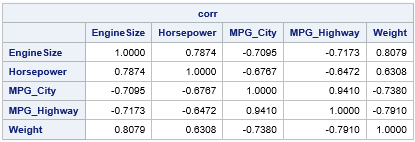The USE and READ statements read SAS data sets. However, there are no SAS data sets in CAS, so these statements are not supported in the iml action. Instead, the iml action supports reading a CAS table into a matrix. Whereas the READ statement in PROC IML reads data sequentially, the iml action reads data from a CAS table in parallel by using multiple threads.

### The rows in a CAS table do not have a defined order

Before showing how to read a CAS table into a matrix, let's discuss a characteristic of CAS tables that might be unfamiliar to you. Namely, the order of observations in a CAS table is undefined.

Recall that one purpose of CAS is to process massive amounts of data. Large data tables might be stored in a distributed fashion across multiple nodes in a cluster. When rows of data are read, they are read by using multiple threads that execute in parallel. A consequence of this fact is that CAS data tables do not have an inherent order. If this sounds shocking, realize that the order of the observations does not matter for most data analysis. For example, order does not affect descriptive statistics such as the mean or percentiles. Furthermore, any analysis that uses the sum-of-squares crossproduct matrix (X`*X) is unaffected by reordering the observations. This includes correlation, regression, and a lot of multivariate statistics (for example, principal component analysis).

Of course, for some analyses (such as time series) and for some matrix computations, the order of rows is important. Therefore, the iml action has a way to ensure that the rows of a matrix are in a specific order. If the CAS table has a variable with the name _ROWID_ (or _ROWORDER_), then the data rows are sorted by that variable before the IML matrix is created. This happens automatically when you use the MatrixCreateFromCAS function, which is discussed in a subsequent section. (The _ROWID_ is used only to sort the rows; it does not become a column in the matrix.)

You can use the iml action to read any CAS table. If you read a table that does not contain a _ROWID_ variable, the order of rows in the data matrix might change from run to run.

### Upload a data set from SAS to CAS

There are several ways to upload a SAS data set into a CAS table. This example uses the DATA step. By using the DATA step, you can also add a _ROWID_ variable to the data, in case you want the rows of the IML matrix to be in the same order as the data set.

I assume you have already established a CAS session. The following statements use the LIBNAME statement in SAS to create a libref to the active caslib in the current CAS session. You can use this libref to upload a data set into a CAS table:

```libname mycaslib cas; /* libref to the active caslib in the current CAS session */ data mycaslib.cars; /* create CAS table named CARS in the active caslib */ set Sashelp.Cars; _ROWID_ + 1; /* add a sort variable */ run;   /* Optional: verify that the CAS table includes the _ROWID_ variable */ proc cas; columnInfo / table='cars'; run;```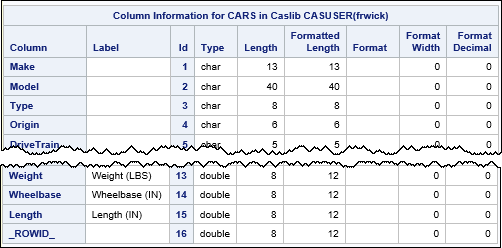The output of the columnInfo action shows that the cars data table was created and includes the _ROWID_ variable.

### How to read a CAS data table in the iml action

To read variables from a CAS data table into a matrix, you can use the CreateMatrixFromCAS function. The syntax of the function is
X = MatrixCreateFromCAS(caslib, TableName, options);
where

• caslib is a caslib that specifies the location of the table. You can specify a blank string if you want to use the default caslib. Or you can specify a caslib as a string. For example, if the CAS table is in your personal caslib, specify 'CASUSER(userName)', where userName is your login name. For more information, see the CAS documentation about caslibs.
• TableName is a string that specifies the name of the CAS table.
• options is string that enables you to read only part of the data. The most common option is the KEEP statement, which you can use to specify all numeric values ('KEEP=_NUMERIC_', which is the default), all character variables ('KEEP=_CHARACTER_'), or to specify certain variables ('KEEP=X1 X1 Y Z').

Recall that matrices in the SAS/IML language are either numeric or character. If you want to read mixed-type data, you can use the TableCreateFromCAS function.

### Read a CAS table in the iml action

In the previous sections, we created a CAS table ('cars') that contains the Sashelp.Cars data. You can use iml action and the MatrixCreateFromCAS function to read that data table into a matrix, as follows:

```proc cas; loadactionset 'iml'; /* load the iml action set (only once per session) */ run;   proc cas; source ReadData; KeepStmt = 'KEEP=EngineSize Horsepower MPG_City MPG_Highway Weight'; X = MatrixCreateFromCas(' ', 'cars', KeepStmt);   corr = corr(X); varNames = {'EngineSize' 'Horsepower' 'MPG_City' 'MPG_Highway' 'Weight'}; print corr[r=varNames c=varNames format=7.4]; endsource; iml / code=ReadData; run;```

The output is identical to the output from PROC IML and is not shown.

The KEEP= option specifies five numeric variables to read. The first argument to the MatrixCreateFromCAS function is a blank string, which means "use the default caslib." Alternatively, you could specify a caslib such as 'CASUSER(userName)'. The matrix X is the data matrix. Because the CARS table contains a _ROWID_ variable, the rows of X are in the same order as the rows of the Sashelp.Cars data set.

After you read the data, you can write standard SAS/IML programs to manipulate or analyze X. For example, I used the CORR function and the PRINT statement to reproduce the results of the previous PROC IML program.

In the next article, I will show how to use the iml action to write a CAS table that contains data in an IML matrix.

The post Read a CAS data table by using the iml action appeared first on The DO Loop.A previous article provides an introduction and overview of the iml action, which is available in SAS Viya 3.5. The article compares the iml action to PROC IML and states that most PROC IML programs can be modified to run in iml action. This article takes a closer look at what a SAS/IML program looks like in the iml action. If you have an existing PROC IML program, how can you modify it to run in the iml action?

### PROC CAS and the SOURCE/ENDSOURCE block

A feature of SAS Viya is that the actions can be called from multiple languages: SAS, Python, R, Lua, etc. I am a SAS programmer, so in my blog, I will use SAS to call actions.

An action (sometimes called a "CAS action") runs by using the SAS Cloud Analytic Services (CAS). The CAS procedure provides a language (called "CASL," for "CAS Language") that enables you to call CAS actions. The documentation for CASL states that "CASL is a scripting language that you use to prepare arguments for execution of CAS actions, submit actions to the CAS server, and then process action results." In short, PROC CAS enables you to "stitch together" a sequence of action calls into a workflow.

PROC CAS supports a SOURCE/ENDSOURCE block, which defines a program that you can submit to an action that supports programming statements. I will use the SOURCE/ENDSOURCE block to define programs for the iml action. To call the iml action by using PROC CAS, you need to do three things:

1. Load the iml action set. Any CAS action set that is not auto-loaded must be loaded before you can use it. It only needs to be loaded once per session.
2. Use the SOURCE/ENDSOURCE block to define the IML program.
3. Call the iml action to run the program. The syntax to call the action is iml / code=ProgramName

In terms of syntax, a typical program in the iml action has the following structure:

```PROC CAS; loadactionset 'iml'; /* load the iml action set */ source ProgramName; < put the IML program here > endsource; iml / code=ProgramName; /* run the program in the iml action */ RUN;```

### Convert a program from PROC IML to the iml action

The following SAS/IML program defines two vectors and computes their inner product. It then computes the variance of the x data vector. Lastly, it centers and scales the data and computes the variance of the standardized data. This program runs in PROC IML:

```PROC IML; c = {1, 2, 1, 3, 2, 0, 1}; /* weights */ x = {0, 2, 3, 1, 0, 2, 2}; /* data */ wtSum = c` * x; /* inner product (weighted sum) */ var1 = var(x); /* variance of original data */ stdX = (x-mean(x)) / std(x); /* standardize data */ var2 = var(stdX); /* variance of standardized data */ print wtSum var1 var2; QUIT;```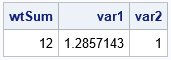This program does not read or write any data sets, which makes it easy to convert to the iml action. The following statements assume that you have a license for the SAS IML product in Viya and that you have already connected to a CAS server.

```/* Example of using PROC CAS in SAS to call the iml action */ PROC CAS; loadactionset 'iml'; /* load the action set (once) */ source pgm; c = {1, 2, 1, 3, 2, 0, 1}; /* weights */ x = {0, 2, 3, 1, 0, 2, 2}; /* data */ wtSum = c` * x; /* inner product (weighted sum) */ var1 = var(x); /* variance of original data */ stdX = (x-mean(x)) / std(x); /* standardize data */ var2 = var(stdX); /* variance of standardized data */ print wtSum var1 var2; endsource; iml / code=pgm; /* call iml action to run the 'pgm' program */ RUN;```

For this example, the SAS/IML statements are exactly the same in both programs. The difference is the way that the program is submitted for execution. For this example, the program is named 'pgm', but you could also have named the program 'MyProgram' or 'StdAnalysis' or 'Robert' or 'Jane'. Whatever identifier you use on the SOURCE statement, use that same identifier for the CODE= parameter when you call in the action.

### Calling the iml action from Python

I use PROC CAS in SAS to call CAS actions. However, I know that Python is a popular programming language for some data scientists, so here is how to call the iml action from Python. You first need to install the SAS Scripting Wrapper for Analytics Transfer (SWAT) package. You can then use the following statements to connect to a CAS server and load the action:

```# Example of using Python to call the iml action import swat # load the swat package s = swat.CAS('myhost', 12345) # use server='myhost'; port=12345 s.loadactionset('iml') # load the action set (once)```

As mentioned earlier, you only need to load the action set one time per session. After the action set is loaded, you can call the iml action by using the following. In Python, triple quotes enable you to preserve the indention and comments in the IML program.

```m = s.iml(code= """ c = {1, 2, 1, 3, 2, 0, 1}; /* weights */ x = {0, 2, 3, 1, 0, 2, 2}; /* data */ wtSum = c` * x; /* inner product (weighted sum) */ var1 = var(x); /* variance of original data */ stdX = (x-mean(x)) / std(x); /* standardize data */ var2 = var(stdX); /* variance of standardized data */ print wtSum var1 var2; """)```

### More realistic examples

In this case, the program did not read or write data. However, a typical IML program reads in data, analyzes it, and optionally writes out the results. This is the first (and most common) difference between a program that runs in PROC IML and a similar program that runs in the iml action. A PROC IML program reads and writes SAS data sets, whereas actions read and write CAS data tables.

The next article shows an example of a PROC IML program that reads and writes SAS data sets. It compares the PROC IML program to an analogous program that runs in the iml action and reads and writes CAS tables.

The post Getting started with the iml action in SAS Viya appeared first on The DO Loop.This article introduces the iml action, which is available in SAS Viya 3.5. The iml action supports most of the same syntax and functionality as the SAS/IML matrix language, which is implemented in PROC IML. With minimal changes, most programs that run in PROC IML also run in the iml action. In addition, the iml action supports new programming features for parallel programming.

Most actions in SAS Viya perform a specific task, but the iml action is different. The iml action provides a set of general programming tools that you can use to implement a custom parallel algorithm. The programmer can control many aspects of the computation, including how the computation is distributed among nodes and threads on a cluster of machines (or threads on a single machine).

Future articles will address the parallel programming capabilities of the iml action. This article provides an overview of the iml action. What is it? How do you get access to it? How is it similar to and different from PROC IML?

### What is the iml action?

Recall that the SAS/IML language is a matrix-vector programming language that supports a rich library of functions in statistics, data analysis, matrix computations, numerical analysis, simulation, and optimization. In SAS 9 ("traditional SAS"), you can access the SAS/IML language by licensing the SAS/IML product and calling the IML procedure. PROC IML is also available in the SAS University Edition.

In SAS Viya 3.5. you get access to the SAS/IML language by licensing the SAS IML product. (Notice that there is no “slash” in the product name.) The SAS IML product gives you access to the iml action and to the IML procedure. Thus, in Viya, you can run all existing PROC IML programs, and you can also write new programs that run in the iml action and use SAS Cloud Analytic Services (CAS).

The iml action belongs to the iml action set. In addition to supporting most of the statements and functions in the SAS/IML language, the iml action supports new functionality that enables you to take advantage of the distributed computational resources in SAS Viya. In particular, you can use the iml action to implement custom parallel algorithms that use multiple nodes and threads on a cluster of machines. Even on one machine, you can run custom parallel programs on a multicore processor.

### How is the iml action similar to PROC IML?

The iml action and the IML procedure share a common syntax. The mathematical and statistical function library is essentially the same in the action and in the procedure. Both environments support arithmetic and linear algebraic operations on matrices, operations to subset and query matrices, and programming features such as writing loops and using IF-THEN/ELSE logic.

Of the 300 functions and statements in the SAS/IML run-time library, only a handful of statements are not supported in the iml action. Most differences are related to the difference between the SAS 9 and SAS Viya environments. PROC IML interacts with traditional SAS constructs (such as data sets and catalogs) and supports calling SAS procedures and interacting with files on your local computer. The iml action interacts with analogous constructs in the Viya environment. It can read and write CAS tables, write analytic stores (astores), and can call other Viya actions.

### Why use the iml action?

The iml action runs on a CAS server. Why might you choose to use the iml action instead of PROC IML? Or convert an existing PROC IML program into the iml action? There are two main reasons:

• You want to use the SAS/IML language as part of a sequence of actions that analyze data that are in CAS tables. By using the iml action, you can read and write CAS tables directly. If you use PROC IML, you need to pull the data from CAS into a SAS data set, run the analysis in PROC IML, and then push the results to a CAS table.
• You want to take advantage of the capabilities of the CAS server to perform parallel processing. You can use the iml action to create custom parallel computations.

### How is the iml action different from PROC IML?

As mentioned previously, the iml action does not support every function and statement that PROC IML supports. The unsupported functions and statements are primarily in four areas:

• Base SAS functions that are not supported in the CAS DATA step. For example, the old random number generator functions RANUNI and RANNOR are not supported in CAS because they cannot generate independent streams of random number in parallel.
• Statements that read or write SAS data sets or text files.
• Functions that create graphics. CAS is for computations. You can download the results of the computation to whatever language you are using to call the CAS actions. For example, I download the results to SAS and create SAS graphs, but you could also use Python or R.
• SAS/IML functions that were deprecated in earlier releases of SAS.

See the documentation for the iml action for a complete list of the PROC IML functions and statements that are not supported in the iml action.

### Will my program run faster in the iml action than PROC IML?

If you take an existing PROC IML program and run it in the iml action, it will take about the same amount of time to run. Sure, it might run a little faster if the machines in your CAS cluster are newer and more powerful than the SAS server or your PC, but that speedup is due to hardware. An existing program does not automatically run faster in the iml action because it runs serially until it encounters a programming statement that can be executed in parallel. There are two main sets of statements that run in parallel:

• Reading and writing data from CAS tables.
• Functions that distribute computations. You, the programmer, need to call these functions to write parallel programs.

So, yes, you can get certain programs to run faster in the iml action, but it doesn't happen automatically. You have to add new input/output statements or call functions that execute tasks in parallel.

### Should you convert from PROC IML to the iml action?

What does this mean for the SAS/IML programmer whose company is changing from SAS 9 to Viya? Do you need to convert hundreds of existing PROC IML programs to run in the iml action? No, absolutely not. As mentioned previously, when you license SAS IML on Viya, you get both PROC IML and the iml action. The existing programs that you wrote in SAS 9 will continue to run in PROC IML in Viya.

The Viya platform provides an opportunity to use the iml action but does not require it. Under what circumstances might you want to convert a program from PROC IML to the iml action? Or write a new program in the iml action instead of using PROC IML? In my opinion, it comes down to two issues: workflow and performance.

• Workflow: If your company is using CAS actions and CAS-enabled procedures, and if your data are stored in CAS tables, then it makes sense to use the iml action instead of the IML procedure. The SAS/IML language is often used to pre- or post-process data for other procedures or actions. The iml action can read and write CAS tables that are created by other CAS actions or that will be consumed by other CAS actions.
• Performance: Suppose that you have a computation that takes a long time to process in PROC IML, but the computation is "embarrassingly parallel." An embarrassingly parallel problem, is one that consists of many identical independent subtasks. The iml action supports several functions for distributing a computation to multiple threads. Examples of embarrassingly parallel computations in statistics and machine learning include Monte Carlo simulation, resampling methods such as the bootstrap, ensemble models, and many "brute force" computations.

I continue this exploration of the iml action in subsequent articles. Related articles include:

• A Getting Started example that shows how to call the iml action and discusses how the action is similar to and different from PROC IML.
• An example that shows how to read and write CAS tables from the iml action.
• The MAPREDUCE function, which enables you to distribute a computation across threads and nodes.
• The PARTASKS function, which enables you to distribute multiple independent computations across threads and nodes.
• The SCORE function, which enables you to evaluate a function in parallel on every row of a CAS table.

For more information and examples, see Wicklin and Banadaki (2020), "Write Custom Parallel Programs by Using the iml Action," which is the basis for these blog posts. Another source is the SAS IML Programming Guide, which includes documentation and examples for the iml action.

The post An introduction to the iml action in SAS Viya appeared first on The DO Loop.